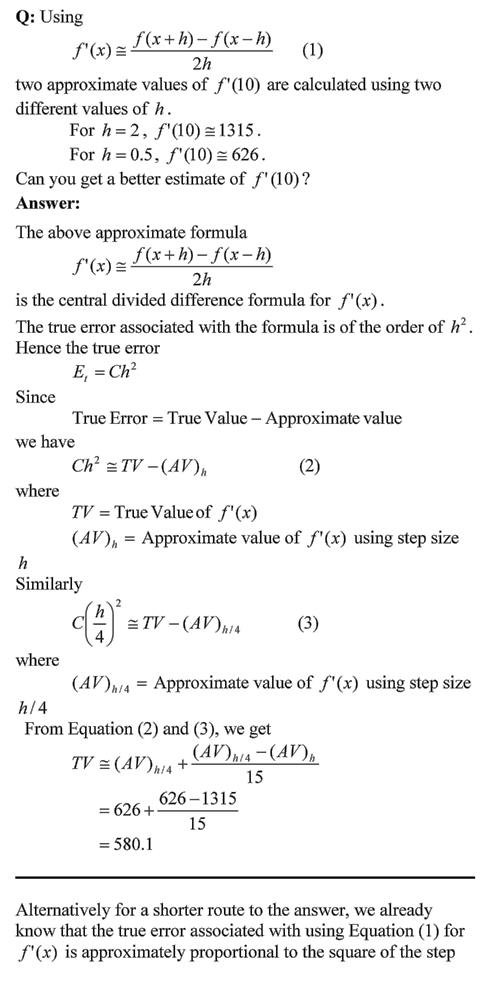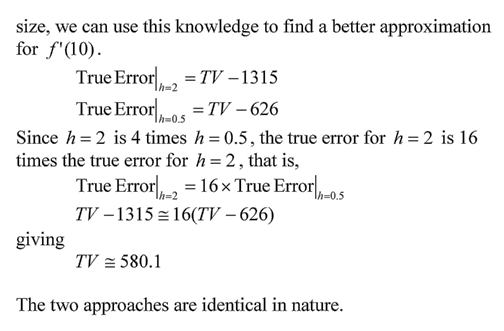# A problem using central divided difference error order

This is a problem I asked in the first examination of my Numerical Methods course in Spring 2009.   The question is that if one gives you an approximate value of the derivative of a function at a certain point using the central divided difference formula for two different step sizes, would you be able to find a better estimate of the derivative?This post is brought to you by Holistic Numerical Methods: Numerical Methods for the STEM undergraduate at http://nm.mathforcollege.com, the textbook on Numerical Methods with Applications available from the lulu storefront, and the YouTube video lectures available at http://www.youtube.com/numericalmethodsguy.

## 0 thoughts on “A problem using central divided difference error order”

1.Nate says:

This strikes me as similar to what is done in Romberg integration–using successive approximations to generate an improved approximation. That also strikes me as a pretty hard question, unless specifically covered in class. How did the students do?

2.Nate says:

This strikes me as similar to what is done in Romberg integration–using successive approximations to generate an improved approximation. That also strikes me as a pretty hard question, unless specifically covered in class. How did the students do?

3.Autar Kaw says:

Yes, it is similar to how Romberg Integration is derived as it also an extrapolation technique to get a better estimate of a numerical answer.

The question is not hard as we spent quite a bit of class time discussing the error in CDD via Taylor series. We then used that discussion to derive the formula for a better estimate from using values when the step size is halved.

4.Autar Kaw says:

Yes, it is similar to how Romberg Integration is derived as it also an extrapolation technique to get a better estimate of a numerical answer.

The question is not hard as we spent quite a bit of class time discussing the error in CDD via Taylor series. We then used that discussion to derive the formula for a better estimate from using values when the step size is halved.

5.Aniruddh says:

HALLO ALL .. I DO HAVE A FUNNY QUESTION TO ASK AS I AM A BEGINNER IN NUMERICAL METHODS… I DO HAVE TO APPEAR FOR AN EXAMINATION IN NUMERICAL METHODS… THERE I DO HAVE A TOPIC OF INTEGRATION…..I WILL TYPE THE SYLLABUS FOR THE SAME … MY PROBLEM IS THAT I FIND THIS SYLLABUS IS VERY BRIEF….IN THIS ONLY FOUR WORDS ARE WRITTEN AND NO BODY IS ABLE TO TELL ME WHAT IS EXPECTED TO STUDY UNDER THIS CHAPTER EXACTLY… KINDLY GUIDE ME WHETHER ALLL THESE 1. NEWTON COTES FORMULAES LIKE SIMSON’S AND TRAPEZODIAL CAN BE TREATED AS DIVIDED DIFFERENCE FORMULAE OR NOT??? WHAT IS MEANT BY “GUASS QUADRATURE FOR DOUBLE AND TRIPPLE INTEGRATION”???

kINDLY GO THROUGH THE SYLLABUS I HAVE TO STUDY FOR
CHAPTER: DIFFERNTIATION AND INTEGRATION
1. DIVIDED DIFFERENCE FORMULAE2. ROMBERG INTEGRATION3. GAUSS QUADRATURE FOR DOUBLE AND TRIPPLE INTEGRATION

6.Aniruddh says:

HALLO ALL .. I DO HAVE A FUNNY QUESTION TO ASK AS I AM A BEGINNER IN NUMERICAL METHODS… I DO HAVE TO APPEAR FOR AN EXAMINATION IN NUMERICAL METHODS… THERE I DO HAVE A TOPIC OF INTEGRATION…..I WILL TYPE THE SYLLABUS FOR THE SAME … MY PROBLEM IS THAT I FIND THIS SYLLABUS IS VERY BRIEF….IN THIS ONLY FOUR WORDS ARE WRITTEN AND NO BODY IS ABLE TO TELL ME WHAT IS EXPECTED TO STUDY UNDER THIS CHAPTER EXACTLY… KINDLY GUIDE ME WHETHER ALLL THESE 1. NEWTON COTES FORMULAES LIKE SIMSON’S AND TRAPEZODIAL CAN BE TREATED AS DIVIDED DIFFERENCE FORMULAE OR NOT??? WHAT IS MEANT BY “GUASS QUADRATURE FOR DOUBLE AND TRIPPLE INTEGRATION”???

kINDLY GO THROUGH THE SYLLABUS I HAVE TO STUDY FOR
CHAPTER: DIFFERNTIATION AND INTEGRATION
1. DIVIDED DIFFERENCE FORMULAE2. ROMBERG INTEGRATION3. GAUSS QUADRATURE FOR DOUBLE AND TRIPPLE INTEGRATION